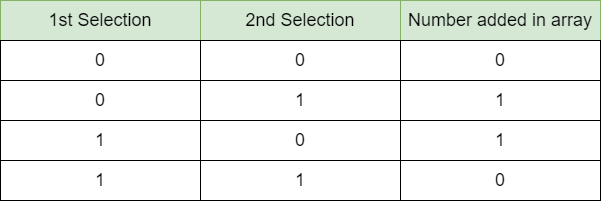GeeksforGeeks App
Open AppBrowser
Continue

# Reduce Binary Array by replacing both 0s or both 1s pair with 0 and 10 or 01 pair with 1

Given a binary array arr[] of size N, the task is to find the last number remaining in the array after performing a set of operations. In each operation, select any two numbers and perform the following:

• If both numbers are the same, remove them from the array and insert a 0.
• If both numbers are different, remove both of them and insert a 1.

Example:

Input: arr[]={0, 0, 1}
Output: 1
Explanation: There are two possible sequence of operations as follows:

• arr[] = {0, 0, 1}, delete (0, 1) and insert 0 => arr[] = {0, 0}, delete (0, 0) and insert 1=> arr[] = {1}.
• arr[] = {0, 0, 1}, delete (0, 0) and insert 0 => arr[] = {0, 1}, delete (0, 1) and insert 1=> arr[] = {1}.

Hence the remaining element is 1.

Input: arr[]={1, 0, 0, 0, 1}
Output: 0

Approach: The given problem can be solved based on the following observations:

• 2 same numbers are getting replaced by a 0.
• 2 different numbers are getting replaced by a 1.

Now, the creating a table for each outcome:Upon careful observation of the above table, it can be noticed that the table represents the bitwise XOR operation. Hence, the remaining integer will be equal to the bitwise XOR of the given array elements which can be further simplified as if the frequency of 1 is even, the result is 0, otherwise, it’s 1.

Below is the implementation of the above approach.

## C++

 `// C++ program of the above approach``#include ``using` `namespace` `std;` `// Function to find last remaining``// integer in the given array``int` `lastNumber(vector<``int``>& arr)``{` `    ``// Variable to store the``    ``// frequency of 1``    ``int` `one = 0;` `    ``// Loop to iterate the``    ``// given array``    ``for` `(``int` `x : arr) {``        ``if` `(x == 1) {``            ``one += 1;``        ``}``    ``}` `    ``// If frequency of 1 is even``    ``if` `(one % 2 == 0)``        ``return` `0;` `    ``// If frequency of 1 is odd``    ``return` `1;``}` `// Driver Code``int` `main()``{``    ``vector<``int``> arr = { 1, 0, 0, 0, 1 };``    ``cout << lastNumber(arr);``}`

## Java

 `// Java program of the above approach``import` `java.util.ArrayList;``class` `GFG {` `  ``// Function to find last remaining``  ``// integer in the given array``  ``static` `Integer lastNumber(ArrayList arr)``  ``{` `    ``// Variable to store the``    ``// frequency of 1``    ``int` `one = ``0``;` `    ``// Loop to iterate the``    ``// given array``    ``for` `(``int` `x : arr) {``      ``if` `(x == ``1``) {``        ``one += ``1``;``      ``}``    ``}` `    ``// If frequency of 1 is even``    ``if` `(one % ``2` `== ``0``)``      ``return` `0``;` `    ``// If frequency of 1 is odd``    ``return` `1``;``  ``}` `  ``// Driver Code``  ``public` `static` `void` `main(String args[]) {``    ``ArrayList arr = ``new` `ArrayList();``    ``arr.add(``1``);``    ``arr.add(``0``);``    ``arr.add(``0``);``    ``arr.add(``0``);``    ``arr.add(``1``);` `    ``System.out.println(lastNumber(arr));``  ``}``}` `// This code is contributed by gfgking`

## Python3

 `# python program of the above approach` `# Function to find last remaining``# integer in the given array``def` `lastNumber(arr):` `    ``# Variable to store the``    ``# frequency of 1``    ``one ``=` `0` `    ``# Loop to iterate the``    ``# given array``    ``for` `x ``in` `arr:``        ``if` `(x ``=``=` `1``):``            ``one ``+``=` `1` `    ``# If frequency of 1 is even``    ``if` `(one ``%` `2` `=``=` `0``):``        ``return` `0` `    ``# If frequency of 1 is odd``    ``return` `1` `# Driver Code``if` `__name__ ``=``=` `"__main__"``:` `    ``arr ``=` `[``1``, ``0``, ``0``, ``0``, ``1``]``    ``print``(lastNumber(arr))` `# This code is contributed by rakeshsahni`

## C#

 `// C# program of the above approach``using` `System;``using` `System.Collections.Generic;` `class` `GFG{` `// Function to find last remaining``// integer in the given array``static` `int` `lastNumber(List<``int``> arr)``{``    ` `    ``// Variable to store the``    ``// frequency of 1``    ``int` `one = 0;` `    ``// Loop to iterate the``    ``// given array``    ``foreach``(``int` `x ``in` `arr)``    ``{``        ``if` `(x == 1)``        ``{``            ``one += 1;``        ``}``    ``}` `    ``// If frequency of 1 is even``    ``if` `(one % 2 == 0)``        ``return` `0;` `    ``// If frequency of 1 is odd``    ``return` `1;``}` `// Driver Code``public` `static` `void` `Main()``{``    ``List<``int``> arr = ``new` `List<``int``>(){ 1, 0, 0, 0, 1 };``    ` `    ``Console.WriteLine(lastNumber(arr));``}``}` `// This code is contributed by ukasp`

## Javascript

 ``

Output

`0`

Time Complexity: O(N)
Auxiliary Space: O(1)

Dynamic Approach:

1. Create a dynamic programming table dp of size N x N, where N is the size of the input array arr[]. Each element dp[i][j] represents the last number remaining in the subarray starting from index i to index j.
2. Initialize the diagonal elements of the dp table to the corresponding elements of the input array arr[].
3. Traverse the subarrays of arr[] in a bottom-up manner, starting from smaller subarrays and building up to the larger subarrays.
4. For each subarray, calculate the value of dp[i][i+len-1] using the following rules:

* If arr[i] == arr[i+len-1], set dp[i][i+len-1] to 0.
* If arr[i] != arr[i+len-1], set dp[i][i+len-1] to 1.

5. After completing the traversal, the remaining element in the entire array is stored in dp[N-1], which represents the                  last number remaining after performing all the operations.

6. Return the value in dp[N-1] as the last remaining number.

Below is the implementation of the above approach:

## C++

 `#include ``#include ` `using` `namespace` `std;` `int` `lastRemainingNumber(vector<``int``>& arr) {``    ``int` `n = arr.size();``    ``vector> dp(n, vector<``int``>(n, 0));` `    ``// Initialize diagonal elements``    ``for` `(``int` `i = 0; i < n; i++) {``        ``dp[i][i] = arr[i];``    ``}` `    ``// Calculate remaining element for each subarray``    ``for` `(``int` `len = 2; len <= n; len++) {``        ``for` `(``int` `i = 0; i <= n - len; i++) {``            ``int` `j = i + len - 1;` `            ``if` `(arr[i] == arr[j]) {``                ``// If both numbers are the same, set the remaining element to 0``                ``dp[i][j] = 0;``            ``} ``else` `{``                ``// If both numbers are different, set the remaining element to 1``                ``dp[i][j] = 1;``            ``}``        ``}``    ``}` `    ``return` `dp[n - 1];``}` `int` `main() {``    ``vector<``int``> arr = {0, 0, 1};``    ``int` `result = lastRemainingNumber(arr);``    ``cout<< result << endl;` `    ``return` `0;``}`

Output

```1
```

Time Complexity: O(N^2)

Auxiliary Space: O(N^2)

My Personal Notes arrow_drop_up